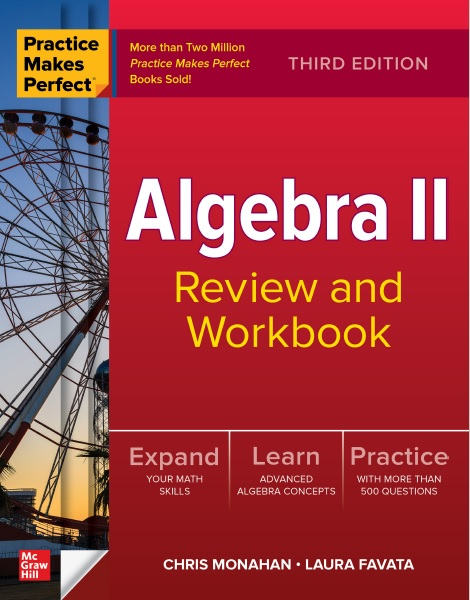# Algebra II: Review and Workbook, Third Edition PDF by Chris Monahan and Laura Favata

## Algebra II: Review and Workbook, Third EditionBy Chris Monahan and Laura Favata

Contents:

Introduction ix

1 Functions: An introduction 1

Relations and inverses 1

Functions 2

Function notation 3

Arithmetic of functions 4

Transformation of functions 6

Inverse of a function 9

Graphical representation of functions 10

2 Linear equations and inequalities 15

Simple linear equations 15

System of linear equations—graphical 18

System of linear equations—substitution 20

System of linear equations—elimination 21

System of linear equations—three variables 23

System of linear equations—matrix 25

System of linear equations—application 27

Linear inequalities 30

System of linear inequalities 31

Absolute value equations 35

Absolute value inequalities 36

The parabola 38

Special factoring formulas 41

Trial and error 42

Completing the square 47

Applications 50

Square root function 52

Circles 53

Ellipses 54

Hyperbolas 56

Systems of equations 59

Powers of i 62

Arithmetic of complex numbers 63

The discriminant and nature of the roots of a quadratic equation 65

Sum and product of roots of a quadratic equation 67

5 Polynomial functions 69

Even and odd functions 69

Inverse functions 71

End behavior 72

Factor theorem 73

6 Rational and irrational functions 75

Rational functions 75

Multiplying and dividing rational expressions 77

Adding and subtracting rational expressions 79

Solving rational equations 82

Irrational functions 84

Simplifying irrational expressions 85

Solving irrational equations 86

7 Exponential and logarithmic functions 89

Properties of exponents 89

Exponential and logarithmic functions 92

Properties of logarithms 95

Solving exponential and logarithmic equations 97

8 Sequences and series 103

Summation notation 103

Recursion 104

Arithmetic sequences 106

Arithmetic series 107

Geometric sequences 108

Geometric series 110

9 Introduction to probability 112

Fundamental theorem of counting 112

Permutations 114

Combinations 116

Binomial expansions 118

Conditional probability 120

Binomial probability/Bernoulli trial 123

10 Introduction to statistics 126

Measures of central tendency 126

Measures of dispersion 129

Normal distribution 134

Regressions 141

11 Inferential statistics 149

Basics 149

Central limit theorem and standard error 150

Standardized (z) scores 153

The basics of inferential statistics 155

Confidence intervals 156

Tests of hypotheses 159

Simulation 162

12 Trigonometry: Right triangles and radian measure 166

Right triangle trigonometry 166

Special right triangles 169

The unit circle: First quadrant 170

The unit circle—beyond the first quadrant 172

Basic trigonometric identities 176

Area of a triangle 179

Law of sines 180

Ambiguous case 182

Law of cosines 185

13 Graphs of trigonometric functions 189

Amplitude and period 189

Graphing trigonometric functions 191

Inverse trigonometric functions 195

Solving trigonometric equations 199